让你的 C 程序更有效率的 10 种方法

CIIS 2019丨CIIS 2019丨倒计时10天丨CIIS 2019 嘉宾阵容公布！>>>本文向你介绍规范你的C代码的10种方法。

1. 避免不必要的函数调用

void str_print( char *str )
{
int i;
for ( i = 0; i < strlen ( str ); i++ ) {
printf("%c",str[ i ] );
}
}

void str_print1 ( char *str )
{
int len;
len = strlen ( str );
for ( i = 0; i < len; i++ ) {
printf("%c",str[ i ] );
}
}

2、避免不必要内存引用

int multiply ( int *num1 , int *num2 )
{
*num1 = *num2;
*num1 += *num2;
return *num1;
}
int multiply1 ( int *num1 , int *num2 )
{
*num1 = 2 * *num2;
return *num1;
}

3、节约内存内存对齐和填充概念

struct {
char c;
int i;
short s;
}str_1;

struct {
char c;
short s;
int i;
}str_2;

4、使用无符号整数，而不是整数的，如果你知道的值将永远是否定的。

有些处理器可以处理无符号整数比有符号整数的运算速度要快。这也是很好实践，帮助self-documenting代码

5、一个逻辑条件语句中常数项永远在左侧。

int x = 4;
if ( x = 1 ) {
x = x + 2;
printf("%d",x);          // Output is 3
}

int x = 4;
if ( 1 = x ) {
x = x + 2;
printf("%d",x);   // Compilation error
}

6、在可能的情况下使用typedef替代macro。当然有时候你无法避免macro，但是typedef更好。

typedef int* INT_PTR;
INT_PTR a , b;
# define INT_PTR int*;
INT_PTR a , b;

7、确保声明和定义是静态的，除非您希望从不同的文件中调用该函数

A）两个或两个以上具有相同名称静态函数可用于在不同的文件

B）编译消耗减少，因为没有外部符号处理

/*first_file.c*/
static int foo ( int a )
{
/*Whatever you want to in the function*/
}
/*second_file.c*/
int foo ( int )
int main()
{
foo();      // This is not a valid function call as the function foo can only be called by any other function within first_file.c where it is defined.
return 0;
}

8、使用Memoization，以避免递归重复计算

Fibonacci斐波那契）问题;Fibonacci问题是可以通过简单递归方法来解决：

int fib ( n )
{
if ( n == 0 || n == 1 ) {
return 1;
}
else {
return fib( n - 2 ) + fib ( n - 1 );
}
}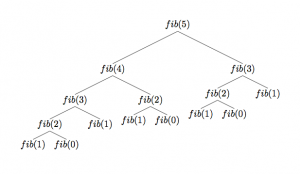fibonacci 函数Memoization的代码，应该是下面的这个样子：

int calc_fib ( int n )
{
int val[ n ] , i;
for ( i = 0; i <=n; i++ ) {
val[ i ] = -1;         // Value of the first n + 1 terms of the fibonacci terms set to -1
}
val[ 0 ] = 1;                 // Value of fib ( 0 ) is set to 1
val[ 1 ] = 1;            // Value of fib ( 1 ) is set to 1
return fib( n , val );
}
int fib( int n , int* value )
{
if ( value[ n ] != -1 ) {
return value[ n ];              // Using memoization
}
else {
value[ n ] = fib( n - 2 , value ) + fib ( n - 1 , value );          // Computing the fibonacci term
}
return value[ n ];                // Returning the value
}

9、避免悬空指针和野指针

void dangling_example()
{
int *dp = malloc ( sizeof ( int ));
/*........*/
free( dp );              // dp is now a dangling pointer
dp = NULL;        // dp is no longer a dangling pointer
}

void wild_example()
{
int *ptr;        // Uninitialized pointer
printf("%u"\n",ptr );
printf("%d",*ptr );
}

10、 永远记住释放你分配给程序的任何内存。上面的例子就是如果释放dp指针（我们使用malloc()函数调用）。

最新评论（17）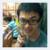4. Use unsigned ints instead of ints if you know the value will never be negative.

4、如果你知道要处理的值是非负数的，使用无符号整数，而不是整数的。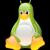int multiply ( int *num1 , int *num2 )
{
*num1 = *num2;
*num1 += *num2;
return *num1;
}
int multiply1 ( int *num1 , int *num2 )
{
*num1 = 2 * *num2;
return *num1;
}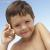int calc_fib ( int n )
{
int val[ n ] , i;
for ( i = 0; i <=n; i++ ) {
val[ i ] = -1; // Value of the first n + 1 terms of the fibonacci terms set to -1
}
......
}这里val[n]是静态数组，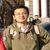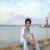int multiply1 ( int *num1 , int *num2 )
{
*num1 = 2 * *num2;
return *num1;
}